NEET  >  31 Years NEET Previous Year Questions: Current Electricity - 1

# 31 Years NEET Previous Year Questions: Current Electricity - 1

Test Description

## 35 Questions MCQ Test Physics Class 12 | 31 Years NEET Previous Year Questions: Current Electricity - 1

31 Years NEET Previous Year Questions: Current Electricity - 1 for NEET 2023 is part of Physics Class 12 preparation. The 31 Years NEET Previous Year Questions: Current Electricity - 1 questions and answers have been prepared according to the NEET exam syllabus.The 31 Years NEET Previous Year Questions: Current Electricity - 1 MCQs are made for NEET 2023 Exam. Find important definitions, questions, notes, meanings, examples, exercises, MCQs and online tests for 31 Years NEET Previous Year Questions: Current Electricity - 1 below.
Solutions of 31 Years NEET Previous Year Questions: Current Electricity - 1 questions in English are available as part of our Physics Class 12 for NEET & 31 Years NEET Previous Year Questions: Current Electricity - 1 solutions in Hindi for Physics Class 12 course. Download more important topics, notes, lectures and mock test series for NEET Exam by signing up for free. Attempt 31 Years NEET Previous Year Questions: Current Electricity - 1 | 35 questions in 70 minutes | Mock test for NEET preparation | Free important questions MCQ to study Physics Class 12 for NEET Exam | Download free PDF with solutions
 1 Crore+ students have signed up on EduRev. Have you?
31 Years NEET Previous Year Questions: Current Electricity - 1 - Question 1

### In the circuit shown, if a conducting wire is connected between points A and B, the current in this wire will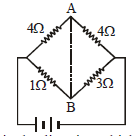Detailed Solution for 31 Years NEET Previous Year Questions: Current Electricity - 1 - Question 1

Current will flow from B to A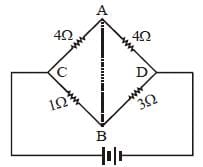Potential drop over the resistance CA will be
more due to higher value of resistance. So
potential at A will be less as compared with at
B. Hence, current will flow from B to A.

31 Years NEET Previous Year Questions: Current Electricity - 1 - Question 2

### Kirchhoff’s first and second laws for electricalcircuits are consequences of 

Detailed Solution for 31 Years NEET Previous Year Questions: Current Electricity - 1 - Question 2

Kirchhoff ' s first law deals with conservation
of electrical charge & the second law deals
with conservation of electrical energy.

31 Years NEET Previous Year Questions: Current Electricity - 1 - Question 3

### Two cells, having the same e.m.f., are connected in series through an external resistance R. Cells have internal resistances r1 and r2 (r1 > r2) respectively. When the circuit is closed, the potential difference across the first cell is zero. The value of R is [2006

Detailed Solution for 31 Years NEET Previous Year Questions: Current Electricity - 1 - Question 3

Current in the circuit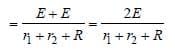P.D. across first cell = E – ir1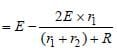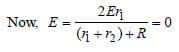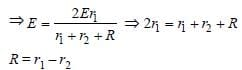31 Years NEET Previous Year Questions: Current Electricity - 1 - Question 4

The total power dissipated in watts in the circuit shown here is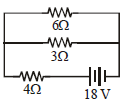Detailed Solution for 31 Years NEET Previous Year Questions: Current Electricity - 1 - Question 4

Power dissipiated = P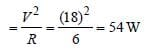31 Years NEET Previous Year Questions: Current Electricity - 1 - Question 5

Three resistances P, Q, R each of 2 Ω  and an unknown resistance S form the four arms of a Wheatstone bridge circuit. When a resistance of 6 Ω is connected in parallel to S the bridge gets balanced. What is the value of S?

Detailed Solution for 31 Years NEET Previous Year Questions: Current Electricity - 1 - Question 5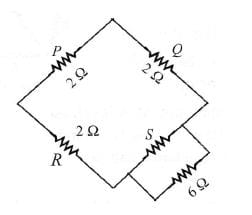For a balanced Wheatstone bridge, we get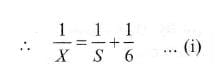Therefore, the equivalent circuit diagram drawn below.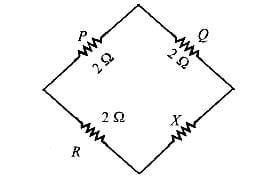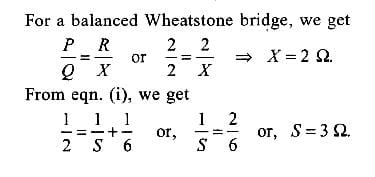31 Years NEET Previous Year Questions: Current Electricity - 1 - Question 6

The resistance of an ammeter is 13 Ω and itsscale is graduated for a current upto 100 amps.After an additional shunt has been connectedto this ammeter it becomes possible to measurecurrents upto 750 amperes by this meter. Thevalue of shunt-resistance is 

Detailed Solution for 31 Years NEET Previous Year Questions: Current Electricity - 1 - Question 6

We know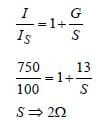31 Years NEET Previous Year Questions: Current Electricity - 1 - Question 7

A steady current of 1.5 amp flows through acopper voltameter for 10 minutes. If the electrochemical equivalent of copper is30 × 10–5 g coulomb–1, the mass of copperdeposited on the electrode will be 

Detailed Solution for 31 Years NEET Previous Year Questions: Current Electricity - 1 - Question 7

We have, m = ZIt
where, Z is the electrochemical equivalent
of copper.
⇒ m = 30 x10-5 x1.5 x10 x 60
= 0.27 gm.

31 Years NEET Previous Year Questions: Current Electricity - 1 - Question 8

A current of 3 amp flows through the 2Ω resistor shown in the circuit. The power dissipated in the 5-Ω resistor is: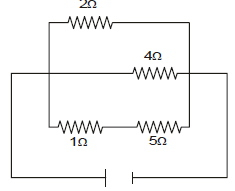Detailed Solution for 31 Years NEET Previous Year Questions: Current Electricity - 1 - Question 8

Clearly, 2Ω, 4Ω and ( 1 + 5) Ωresistors are
in parallel. Hence, potential difference is
same across each of them.
∴ I1 × 2 = I2 × 4 = I3 × 6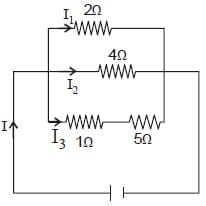Given I1 = 3A ∴ I1 × 2 = I3 × 6
Given I1 = 3A.
∴ I1 × 2 = I3 × 6 providesNow, the potential across the 5Ω resistor is
V = I3 × 5 = 1 × 5 = 5V.
∴ the power dissipated in the 5Ωresistor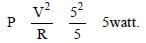31 Years NEET Previous Year Questions: Current Electricity - 1 - Question 9

A wire of a certain material is stretched slowlyby ten per cent. Its new resistance and specificresistance become respectively: 

Detailed Solution for 31 Years NEET Previous Year Questions: Current Electricity - 1 - Question 9

In stretching, specific resistance remains unchanged.
After stretching, specific resistance (rho) will remain same.
Original resistance of the wire,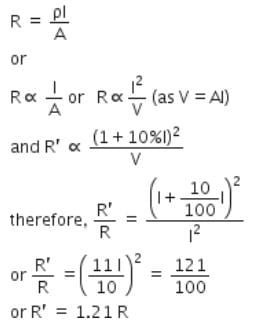31 Years NEET Previous Year Questions: Current Electricity - 1 - Question 10

A cell can be balanced against 110 cm and 100cm of potentiometer wire, respectively with andwithout being short circuited through aresistance of 10Ω. Its internal resistance is 

Detailed Solution for 31 Years NEET Previous Year Questions: Current Electricity - 1 - Question 10

Here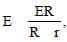hence the lengths 110 cm
and 100 cm are interchanged.
Without being short-circuited through R,
only the battery E is balanced.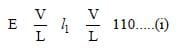When R is connected across E, Ri, V/L, l2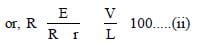Dividing (i) by (ii), we get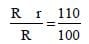or, 100 R + 100 r = 110 R
or, 10 R = 100 r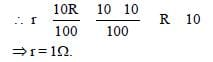31 Years NEET Previous Year Questions: Current Electricity - 1 - Question 11

A galvanometer of resistance 50 Ω is connectedto battery of 3V along with a resistance of 2950 Ωin series. A full scale deflection of 30 divisions isobtained in the galvanometer. In order to reducethis deflection to 20 divisions, the resistance inseries should be 

Detailed Solution for 31 Years NEET Previous Year Questions: Current Electricity - 1 - Question 11

Total internal resistance = (50+2950)Ω
= 3000 Ω
Emf of the cell, ε = 3V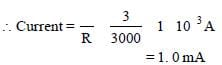Current for full scale deflection of 30
divisions is 1.0 mA.
∴ Current for a deflection of 20 divisions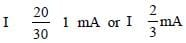Let the resistance be x Ω. Then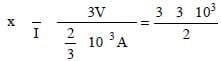= 4500 Ω
But the resistance of the galvanometer
is 50Ω
= (4500 –50) Ω= 4450 Ω

31 Years NEET Previous Year Questions: Current Electricity - 1 - Question 12

See the electric circuit shown in the figure.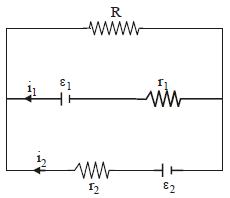Which of the following equations is a correct equation for it? 

Detailed Solution for 31 Years NEET Previous Year Questions: Current Electricity - 1 - Question 12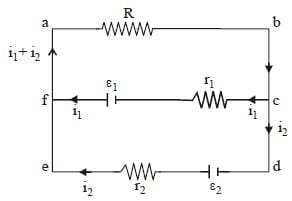Applying Kirchhoff ’s rule in loop abcfa
ε1 – (i1 + i2) R – i1 r1 = 0.

31 Years NEET Previous Year Questions: Current Electricity - 1 - Question 13

A wire of resistance 12 Ωm-1 is bent to form a complete circle of radius 10 cm. The resistance between its two diametrically opposite point, A and B as shown in the figure, is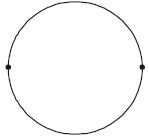Detailed Solution for 31 Years NEET Previous Year Questions: Current Electricity - 1 - Question 13

Total resistance of wire = 12Ω×2π×10−1
= 2.4π
Resistance of each half = 2.4π/2​=1.2π
and as about diameter both parts are in parallel
Req. = 1.2π/2​=0.6πΩ
The correct answer is option D.

31 Years NEET Previous Year Questions: Current Electricity - 1 - Question 14

A student measures the terminal potentialdifference (V) of a cell (of emf E and internalresistance r) as a function of the current (I)flowing through it. The slope and intercept, ofthe graph between V and I, then, respectively,equal: 

Detailed Solution for 31 Years NEET Previous Year Questions: Current Electricity - 1 - Question 14

The terminal potential difference of a cell is
given by V + Ir = E

V= VA – VB
or V = E – Ir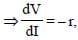Also for, i = 0 then V = E

∴ slope = – r, intercept = E

31 Years NEET Previous Year Questions: Current Electricity - 1 - Question 15

A potentiometer circuit is set up as shown. The potential gradient, across the potentiometer wire, is k volt/cm and the ammeter, present in the circuit, reads 1.0 A when two way key is switched off. The balance points, when the key between the terminals (i) 1 and 2 (ii) 1 and 3, is plugged in, are found to be at lengths ℓ1 cm and ℓ2 cm respectively. The magnitudes, of the resistors R and X, in ohms, are then, equal, respectively, to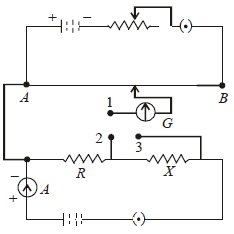Detailed Solution for 31 Years NEET Previous Year Questions: Current Electricity - 1 - Question 15

(i) When key between the terminals 1
and 2 is plugged in,
P.D. across R = IR = k l1
⇒ R = k l1 as I = 1A
(ii) When key between terminals 1 and 3 is
plugged in,
P.D. across (X + R) = I(X + R) = k l2
⇒ X + R = k l2
∴ X = k (l2 – l1)
∴ R = kl1 and X = k (l2 – l1)

31 Years NEET Previous Year Questions: Current Electricity - 1 - Question 16

In producing chlorine by electrolysis 100 kWpower at 125 V is being consumed. How muchchlorine per minute is liberated? (E.C.E. ofchlorine is 0.367×10–6 kg / C) 

Detailed Solution for 31 Years NEET Previous Year Questions: Current Electricity - 1 - Question 16

Power = 100KW
Voltage = 125V
Power = Vi
100×103 = 125i
i = 100000/125
i = 800A
We know that first law of electrolysis
m = Zit (Z = E.C.E)
= 0.367/1000×10-6×800×60
= 367×48×10-6
= 17616×106 kg
= 17.6×10-3 kg

31 Years NEET Previous Year Questions: Current Electricity - 1 - Question 17

Consider the following two statements:
(a) Kirchhoff's junction law follows from the conservation of charge.
(b) Kirchhoff's loop law follows from the conservation of energy.

Which of the following is correct? 

Detailed Solution for 31 Years NEET Previous Year Questions: Current Electricity - 1 - Question 17

Junction law follows from conservation
of charge and loop law is the conservation
of energy

31 Years NEET Previous Year Questions: Current Electricity - 1 - Question 18

The thermo e.m.f E in volts of a certain thermocouple is found to vary with temperature difference θ in °C between the two junctions according to the relation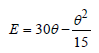The neutral temperature for the thermocouplewill be

Detailed Solution for 31 Years NEET Previous Year Questions: Current Electricity - 1 - Question 18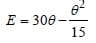For neutral temperature,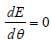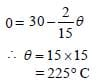Hence, neutral temperature is 225°C.

31 Years NEET Previous Year Questions: Current Electricity - 1 - Question 19

A current of 2A flows through a 2Ω resistorwhen connected across a battery. The samebattery supplies a current of 0.5 A whenconnected across a 9Ω resistor. The internalresistance of the battery is 

Detailed Solution for 31 Years NEET Previous Year Questions: Current Electricity - 1 - Question 19

Let the internal resistance of the battery be
r. Then the current flowing through the
circuit is given by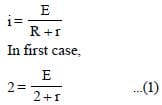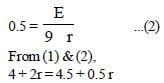⇒ 1.5 r  = 0.5 ⇒ r = 1/3Ω

31 Years NEET Previous Year Questions: Current Electricity - 1 - Question 20

If power dissipated in the 9-Ω resistor in the circuit shown is 36 watt, the potential difference across the 2-Ω resistor is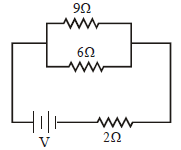Detailed Solution for 31 Years NEET Previous Year Questions: Current Electricity - 1 - Question 20

We have,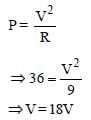Current passing through the 9Ω resistor is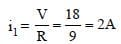The 9Ω and 6Ω resistors are in parallel,
therefore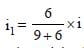where i is the current delivered by the
battery.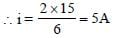Thus, potential difference across 2Ω
resistor is
V = iR
= 5 × 2
= 10V

31 Years NEET Previous Year Questions: Current Electricity - 1 - Question 21

The rate of increase of thermo–e.m.f. withtemperature at the neutral temperature of athermocouple 

Detailed Solution for 31 Years NEET Previous Year Questions: Current Electricity - 1 - Question 21

We have,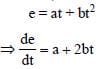At neutral temperature,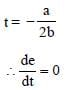31 Years NEET Previous Year Questions: Current Electricity - 1 - Question 22

A thermocouple of negligible resistanceproduces an e.m.f. of 40 μV/°C in the linear rangeof temperature. A galvanometer of resistance 10ohm whose sensitivity is 1μA/div, is employedwith the termocouple. The smallest value oftemperature difference that can be detected bythe system will be [2011M]

Detailed Solution for 31 Years NEET Previous Year Questions: Current Electricity - 1 - Question 22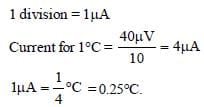31 Years NEET Previous Year Questions: Current Electricity - 1 - Question 23

In the circuit shown in the figure, if the potential at point A is taken to be zero, the potential at point B is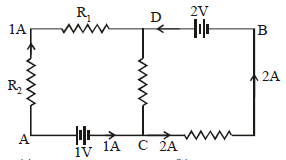Detailed Solution for 31 Years NEET Previous Year Questions: Current Electricity - 1 - Question 23

Current from D to C = 1A
∴ VD – VC = 2 × 1 = 2V
VA = 0     ∴ VC = 1V, ∴ VD – VC = 2
⇒VD – 1 = 2     ∴ VD = 3V
∴ VD – VB = 2 ∴ 3 – VB = 2 ∴ VB = 1V

31 Years NEET Previous Year Questions: Current Electricity - 1 - Question 24

A milli voltmeter of 25 milli volt range is to beconverted into an ammeter of 25 ampere range.The value (in ohm) of necessary shunt will be :

Detailed Solution for 31 Years NEET Previous Year Questions: Current Electricity - 1 - Question 24

Galvanometer is converted into ammeter,
by connected a shunt, in parallel with it.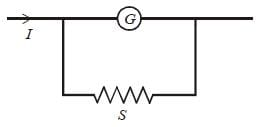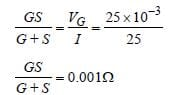Here S << G so
S = 0.001 Ω

31 Years NEET Previous Year Questions: Current Electricity - 1 - Question 25

In the circuit shown the cells A and B have negligible resistances. For VA = 12V, R1 = 500Ω and R = 100Ω the galvanometer (G) shows no deflection. The value of VB is :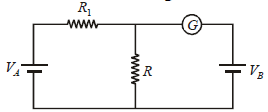Detailed Solution for 31 Years NEET Previous Year Questions: Current Electricity - 1 - Question 25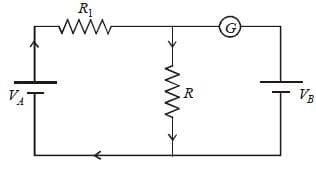Since deflection in galvanometer is zero so
current will flow as shown in the above
diagram.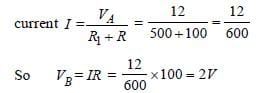31 Years NEET Previous Year Questions: Current Electricity - 1 - Question 26

If voltage across a bulb rated 220 Volt-100 Wattdrops by 2.5% of its rated value, the percentageof the rated value by which the power woulddecrease is : 

Detailed Solution for 31 Years NEET Previous Year Questions: Current Electricity - 1 - Question 26

Resistance of bulb is constant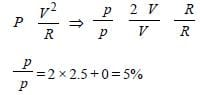31 Years NEET Previous Year Questions: Current Electricity - 1 - Question 27

A ring is made of a wire having a resistance R0 = 12 Ω. Find the points A and B as shown in the figure, at which a current carrying conductor should be connected so that the resistance R of the sub-circuit between these points is equal to 8/3 Ω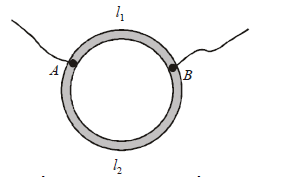Detailed Solution for 31 Years NEET Previous Year Questions: Current Electricity - 1 - Question 27

Let x is the resistance per unit length then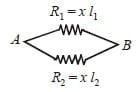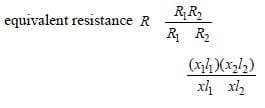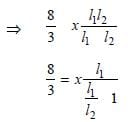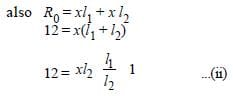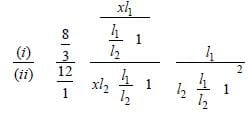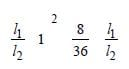(y2 + 1 + 2y) ×  8/36 y  (where  y l1/l2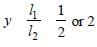31 Years NEET Previous Year Questions: Current Electricity - 1 - Question 28

The power dissipated in the circuit shown in the figure is 30 Watts. The value of R is: [2012M]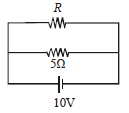Detailed Solution for 31 Years NEET Previous Year Questions: Current Electricity - 1 - Question 28

The power dissipated in the circuit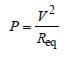v = 10 volt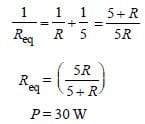Substituting the values in equation (i)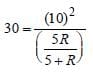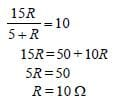31 Years NEET Previous Year Questions: Current Electricity - 1 - Question 29

Cell having an emf ε and internal resistance r is connected across a variable external resistance R. As the resistance R is increased, the plot of potential difference V across R is given by : [2012M]

Detailed Solution for 31 Years NEET Previous Year Questions: Current Electricity - 1 - Question 29

The current through the resistance R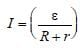The potential difference across R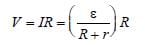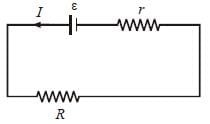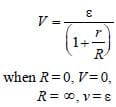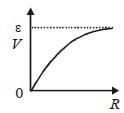Thus V increases as R increases upto certain
limit, but it does not increase further.

31 Years NEET Previous Year Questions: Current Electricity - 1 - Question 30

A wire of resistance 4 Ω is stretched to twice itsoriginal length. The resistance of stretched wirewould be [NEET 2013]

Detailed Solution for 31 Years NEET Previous Year Questions: Current Electricity - 1 - Question 30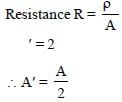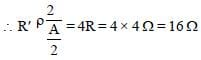Therefore the resistance of new wire
becomes 16 Ω

31 Years NEET Previous Year Questions: Current Electricity - 1 - Question 31

The internal resistance of a 2.1 V cell which givesa current of 0.2 A through a resistance of 10 Ω is[NEET 2013]

Detailed Solution for 31 Years NEET Previous Year Questions: Current Electricity - 1 - Question 31

Given : emf ε = 2.1 V
I = 0.2 A, R = 10Ω
Internal resistance r = ?
From formula.
ε – Ir = V = IR
2.1 – 0.2r = 0.2 × 10
2.1 – 0.2 r = 2 or 0.2 r = 0.1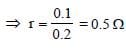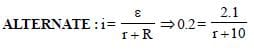⇒ 2.1 = 0.2r +2 ⇒ r = 1/2 = 0.5Ω

31 Years NEET Previous Year Questions: Current Electricity - 1 - Question 32

The resistance of the four arms P, Q, R and S in a Wheatstone’s bridge are 10 ohm, 30 ohm, 30 ohm and 90 ohm, respectively. The e.m.f. and internal resistance of the cell are 7 volt and 5 ohmrespectively. If the galvanometer resistance is50 ohm, the current drawn from the cell will be [NEET 2013]

Detailed Solution for 31 Years NEET Previous Year Questions: Current Electricity - 1 - Question 32

Given : V = 7 V
r = 5Ω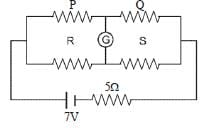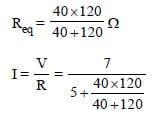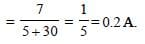31 Years NEET Previous Year Questions: Current Electricity - 1 - Question 33

A 12 cm wire is given a shape of a right angled triangle ABC having sides 3 cm, 4 cm and 5 cm as shown in the figure. The resistance between two ends (AB, BC, CA) of the respective sides are measured one by one by a multi-meter. The resistances will be in the ratio of    [NEET Kar. 2013]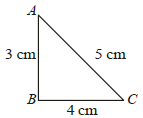Detailed Solution for 31 Years NEET Previous Year Questions: Current Electricity - 1 - Question 33

Resistance is directly proportional to length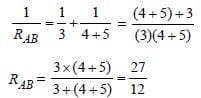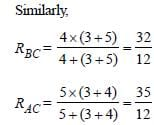∴ RAB : RBC : RAC = 27 : 32 : 35

31 Years NEET Previous Year Questions: Current Electricity - 1 - Question 34

Two rods are joined end to end, as shown. Both have a cross-sectional area of 0.01 cm2. Each is 1 meter long. One rod is of copper with a resistivity of 1.7 × 10–6 ohm-centimeter, the other is of iron with a resistivity of 10–5 ohmcentimeter.     [NEET Kar. 2013]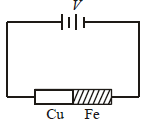How much voltage is required to produce a current of 1 ampere in the rods?

Detailed Solution for 31 Years NEET Previous Year Questions: Current Electricity - 1 - Question 34

Copper rod and iron rod are joined in series.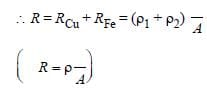From ohm’s law V = RI

= (1.7 × 10–6 × 10–2 + 10–5 × 10–2) ÷ 0.01 × 10–4 volt
= 0.117 volt ( I = 1A)

31 Years NEET Previous Year Questions: Current Electricity - 1 - Question 35

Ten identical cells connected in series areneeded to heat a wire of length one meter andradius ‘r’ by 10ºC in time ‘t’. How many cells willbe required to heat the wire of length two meterof the same radius by the same temperature intime ‘t’? [NEET Kar. 2013]

Detailed Solution for 31 Years NEET Previous Year Questions: Current Electricity - 1 - Question 35

Resistance is directly proportionl to length
of the wire. As length is doubled so mass is
doubled and resistance is doubled.
We have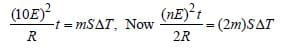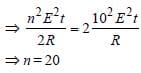## Physics Class 12

157 videos|452 docs|213 tests
 Use Code STAYHOME200 and get INR 200 additional OFF Use Coupon Code
Information about 31 Years NEET Previous Year Questions: Current Electricity - 1 Page
In this test you can find the Exam questions for 31 Years NEET Previous Year Questions: Current Electricity - 1 solved & explained in the simplest way possible. Besides giving Questions and answers for 31 Years NEET Previous Year Questions: Current Electricity - 1, EduRev gives you an ample number of Online tests for practice

## Physics Class 12

157 videos|452 docs|213 tests

### How to Prepare for NEET

Read our guide to prepare for NEET which is created by Toppers & the best Teachers